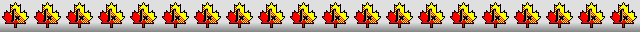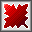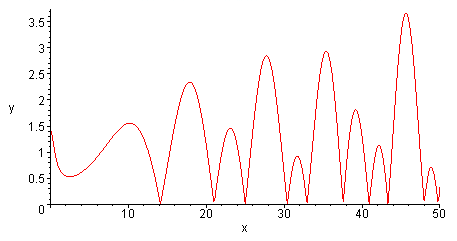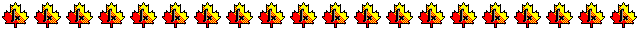Maple worksheets on polynomial interpolationNumerical methods topics:

They are all compatible with Classic Worksheet Maple 10.Introduction - interp.mws

• An introduction to polynomial interpolation: finding the coefficients of an interpolating polynomial by solving a system of equations.

The Lagrange form of the interpolating polynomial - lagrange.mws

• Construction of the Lagrange interpolating polynomial.
• A procedure for constructing the Lagrange interpolating polynomial: lagrange_interp

Working with interpolating polynomials - interp2.mws

• Evaluation of polynomials in nested form: convert( . . , horner).
• Constructing functions from expressions using unapply.
• The Maple procedures map and zip.
• Constructing interpolating polynomials with interp.

The accuracy of an interpolating polynomial - rungexamp.mws

• Investigations involving the Runge example.

Interpolation with unevenly spaced interpolation points - chebyshev.mws

• Description of Chebyshev polynomials.
• Constructing interpolating polynomials with spacing given by the zeros of Chebyshev polynomials.

The Newton interpolation formula - divdiff.mws

• Divided differences.
• The Newton divided differences interpolation formula.
• A procedure for constructing a Newton interpolating polynomial: newton_interp

Using interpolating polynomials to approximate functions - interpoly.mws

• A procedure constructing an interpolating polynomial approximation - interpoly
• interpoly: examples with evenly spaced nodes
• Using an interpolating polynomial to emulate a finite Chebyshev series
• interpoly: general examples

Inverse interpolation and root-finding - invinterp.mws

• Constructing an interpolating polynomial to provide a local inverse for a function, and using it to estimate a zero of the function.
• An iterative root-finding scheme which uses inverse interpolation.
• A procedure for graphing successive steps of the inverse interpolation method: interproot_step
• A procedure implementing a root-finding method which uses inverse interpolation: interproot.

Root-finding for functions of a complex variable - zeta.mws

• Iterative methods of root-finding for functions of a complex variable.
• Finding zeros of the Riemann zeta function.

Function approximation and interpolation procedures - fcnapprx.zipTop of page

Main index